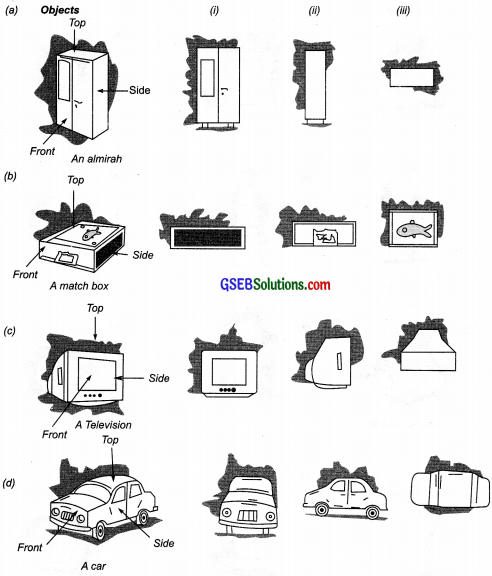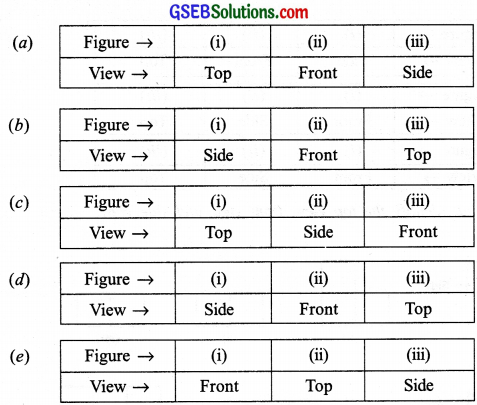# GSEB Solutions Class 8 Maths Chapter 10 Visualizing Solid Shapes Ex 10.1

Gujarat Board GSEB Textbook Solutions Class 8 Maths Chapter 10 Visualizing Solid Shapes Ex 10.1 Textbook Questions and Answers.

## Gujarat Board Textbook Solutions Class 8 Maths Chapter 10 Visualizing Solid Shapes Ex 10.1

Question 1.
For each of the given solid, the two views are given. Match for each solid the corresponding top and front views. The first one is done for you?Solution:
(a) → (iii) → (iv)
(b) → (i) → (v)
(c) → (iv) → (ii)
(d) → (v) → (iii)
(e) → (ii) → (i)Question 2.
For each of the given solid, the three views are given. Identify for each solid the corresponding top, front and side views?Solution:Question 3.
For each given solid, identify the top view, front view and side view?Solution:Question 4.
Draw the front view, side view and top view of the given objects?Solution: